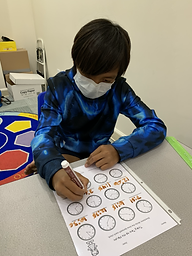## Ms. Kayla

### Target 1​

###### Lesson Type:

Review

Geometry

:

Coordinates

Use coordinates to find a location on a grid.

###### 1:

Identify the x- and y-axis on a coordinate plane.

###### 2:

Locate points in all four quadrants of the coordinate plane.

###### 3:

Recognize which quadrant a point is in, based on the properties of its coordinate pairs.

###### 4:

Understand that the first number in a coordinate pair indicates placement on the x-axis and the second indicates placement on the y-axis.

###### 5:

Graph points in the first and second quadrant of the coordinate plane.

5th

###### Vocabulary:

Coordinate pair, Quadrant, Negative, Positive, X-Axis, Y-Axis

Activities:

1. Students started with identifying the coordinate pairs for points placed on the coordinate plane. .Studebts identified which line represented the x- and y-axis

2. Then students practiced plotting points on a 4-quadrant plane to uncover an image.

3. Lastly, students discovered the properties of coordinate pairs as they placed them on a 4-qudrant plane.### Home Exploration

###### Guiding Questions:## Absent Students:

### Target 2

:

###### 1:

Understand that time is the duration of an event from beginning to end.

###### 2:

Understand that elapsed time can be found by find the total amount of time that passes between the starting and ending time of an event.

4th

###### Vocabulary:

Time, Analog clock, Minute, Hour, Duration

Activities:

1. Students reviewed telling time to the minute on an analog clock.

2. Students were given a schedule and had to determine the beginning, ending, or duration of each activity.### Home Exploration

###### Guiding Questions:### Target 3

:

###### Vocabulary:

Activities:### Home Exploration